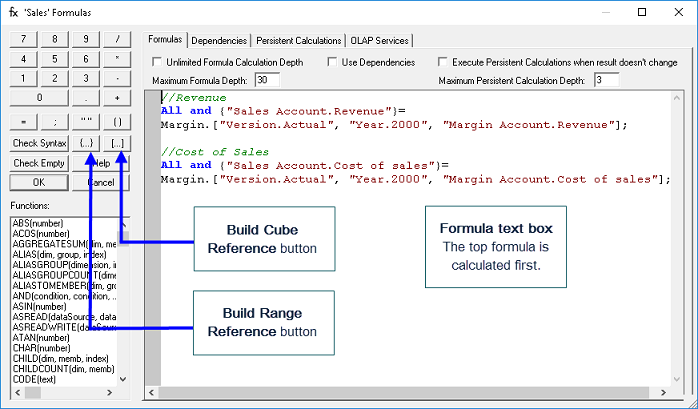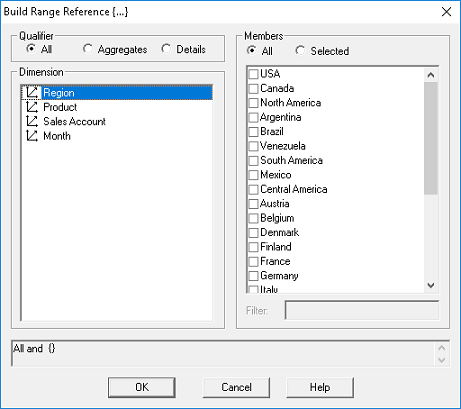Creating Cube FormulasGeneral Procedure

The following is the general procedure for creating Cube formulas in the Formulas dialog box, which we will practice on the next several pages by using the Build Range Reference and Build Cube Reference dialog boxes.

1.   In the PowerOLAP ribbon, select Model tab and Cubes command.
The Cubes dialog box is opened.

2.   Double-click the on the <Cube name>
OR
single-click on a <Cube name> and click the Formulas button.
The Formulas dialog box appears:3.   Type the <formula expression> in the Formulas text box or use the Build Range and/or Cube Reference dialog boxes to define the formula.

4.   Click OK. You are returned to the Cubes dialog box.

5.   Click OK to return to the main application window.

Build Range and Build Cube Reference Dialogs

PowerOLAP helps you build formulas using the Build Range Reference and Build Cube Reference dialog boxes, for the LHS and RHS respectively. The wizard for each is accessed using the following two Define Formula dialog buttons:

 Button Description Build Range ReferenceAllows you to build a Range Reference for the left-hand side (LHS) of a formula (where data will appear). Build Cube ReferenceAllows you to build a Cube Reference for the right-hand side (RHS) of the formula (where data to be calculated by the formula will appear).Figure above: Screenshot of Build Range Reference dialogFigure above: Screenshot of Build Cube Reference dialog

Note that both wizards allow you to specify in great detail where data will appear in a Cube, on the LHS (including whether affected Members are Detail, Aggregate or All); and, assuming the RHS is not a constant, precisely what data is to be “operated on” in an originating Cube (may be the same Cube)

We will proceed with two examples: two different formulas that demonstrate how one might calculate the Margin % (a newly added Member of the Sales Account dimension) in the Sales Cube. The first example concerns calculating Margin % based on the data we already have in the Sales cube—we will refer to this as an “internal cube formula.” The second example is a cross-cube formula, making Margin % in the Sales cube equal to Margin % in the Margin cube where it is already calculated to equal Margindivided by Revenue.